### Reverse-engineering the mechanical Bendix Central Air Data Computer

How did fighter planes in the 1950s perform calculations before compact digital computers were available? The Bendix Central Air Data Computer (CADC) is an electromechanical analog computer that used gears and cams for its mathematics. It was used in military planes such as the F-101 and the F-111 fighters, and the B-58 bomber to compute airspeed, Mach number, and other "air data".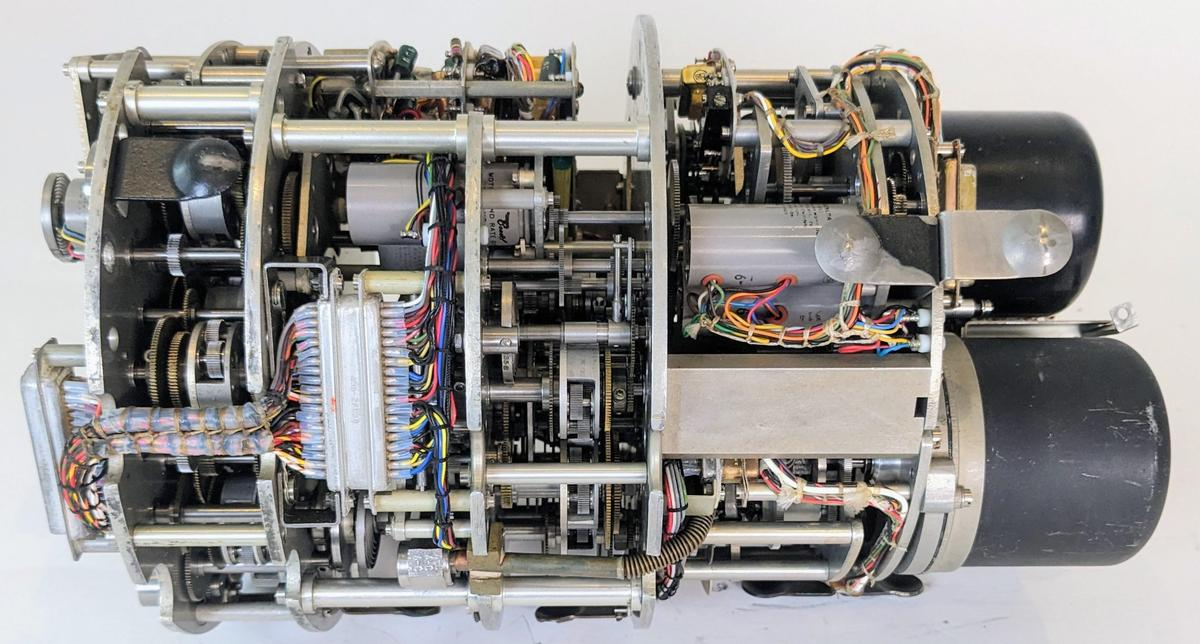The Bendix MG-1A Central Air Data Computer with the case removed, showing the compact gear mechanisms inside. Click this image (or any other) for a larger version.

Aircraft have determined airspeed from air pressure for over a century. A port in the side of the plane provides the static air pressure,1 the air pressure outside the aircraft. A pitot tube points forward and receives the "total" air pressure, a higher pressure due to the speed of the airplane forcing air into the tube. The airspeed can be determined from the ratio of these two pressures, while the altitude can be determined from the static pressure.

But as you approach the speed of sound, the fluid dynamics of air changes and the calculations become very complicated. With the development of supersonic fighter planes in the 1950s, simple mechanical instruments were no longer sufficient. Instead, an analog computer calculated the "air data" (airspeed, air density, Mach number, and so forth) from the pressure measurements. This computer then transmitted the air data electrically to the systems that needed it: instruments, weapons targeting, engine control, and so forth. Since the computer was centralized, the system was called a Central Air Data Computer or CADC, manufactured by Bendix and other companies.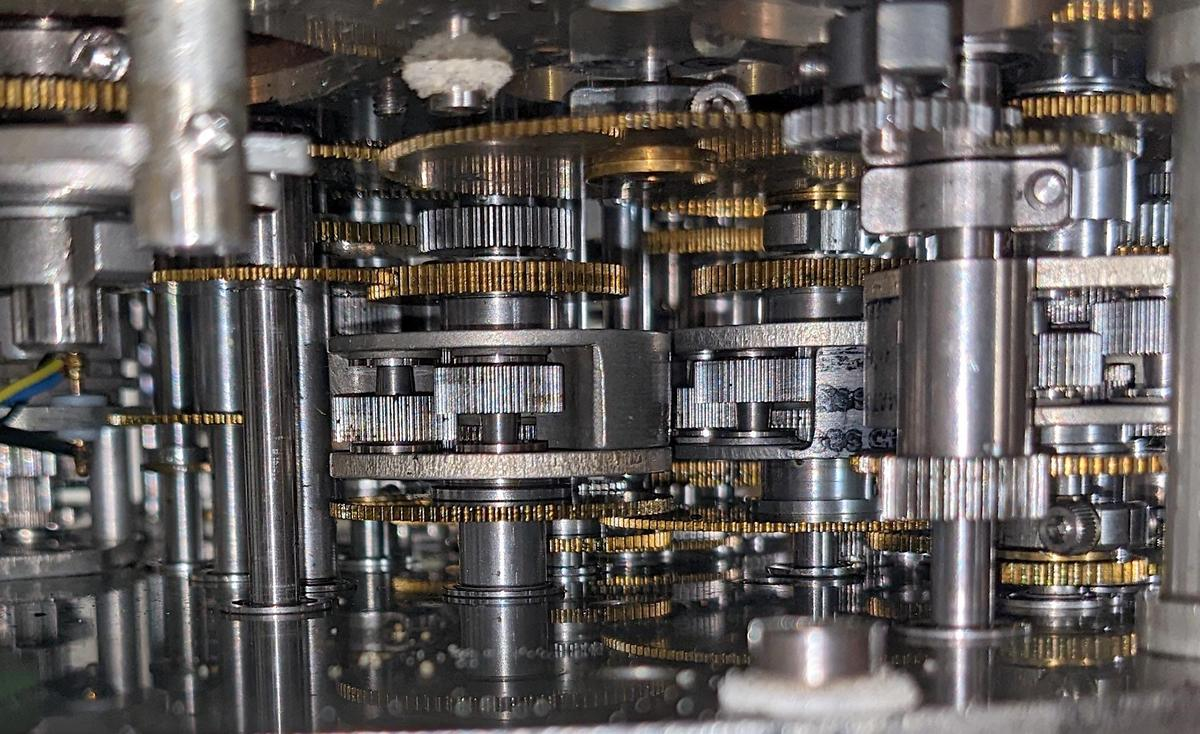A closeup of the numerous gears inside the CADC. Three differential gear mechanisms are visible.

Each value in the CADC is indicated by the rotational position of a shaft. Compact electric motors rotated the shafts, controlled by magnetic amplifier servos. Gears, cams, and differentials performed computations, with the results indicated by more rotations. Devices called synchros converted the rotations to electrical outputs that controlled other aircraft systems. The CADC is said to contain 46 synchros, 511 gears, 820 ball bearings, and a total of 2,781 major parts (but I haven't counted). These components are crammed into a compact cylinder: 15 inches long and weighing 28.7 pounds.

The equations computed by the CADC are impressively complicated. For instance, one equation is:2

$~~~\frac{P_t}{P_s} = \frac{166.9215M^7}{( 7M^2-1)^{2.5}}$

It seems incredible that these functions could be computed mechanically, but three techniques make this possible. The fundamental mechanism is the differential gear, which adds or subtracts values. Second, logarithms are used extensively, so multiplications and divisions become additions and subtractions performed by a differential, while square roots are calculated by gearing down by a factor of 2. Finally, specially-shaped cams implement functions: logarithm, exponential, and functions specific to the application. By combining these mechanisms, complicated functions can be computed mechanically, as I will explain below.

### The differential

The differential gear assembly is the mathematical component of the CADC, as it performs addition or subtraction. The differential takes two input rotations and produces an output rotation that is the sum or difference of these rotations.3 Since most values in the CADC are expressed logarithmically, the differential computes multiplication and division when it adds or subtracts its inputs.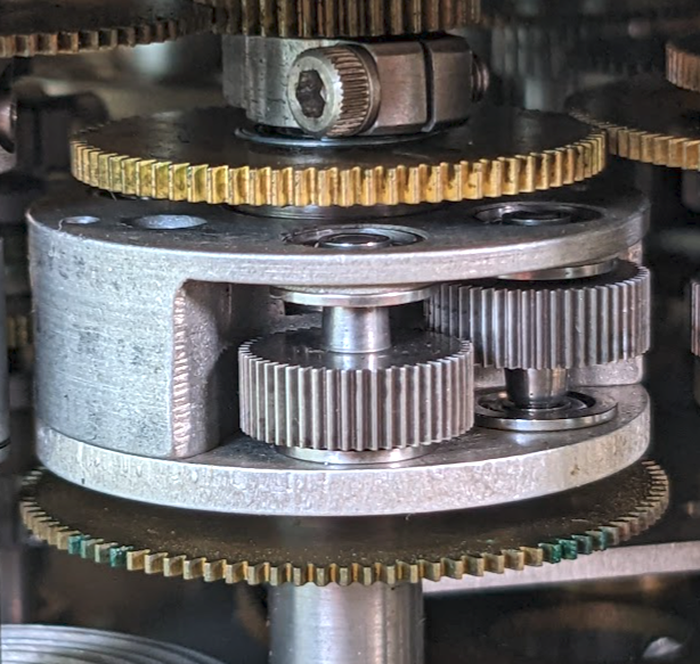A closeup of a differential mechanism.

While the differential functions like the differential in a car, it is constructed differently, with a spur-gear design. This compact arrangement of gears is about 1 cm thick and 3 cm in diameter. The differential is mounted on a shaft along with three co-axial gears: two gears provide the inputs to the differential and the third provides the output. In the photo, the gears above and below the differential are the input gears. The entire differential body rotates with the sum, connected to the output gear at the top through a concentric shaft. (In practice, any of the three gears can be used as the output.) The two thick gears inside the differential body are part of the mechanism.

Note that multiplying a rotation by a constant factor doesn't require a differential; it can be done simply with the ratio between two gears. (If a large gear rotates a small gear, the small gear rotates faster according to the size ratio.) Adding a constant to a rotation is even easier, just a matter of defining what shaft position indicates 0. For this reason, I will ignore constants in the equations.

### The cams

The CADC uses cams to implement various functions. Most importantly, cams compute logarithms and exponentials. Cams also implement complicated functions of one variable such as ${M}/{\sqrt{1 + .2 M^2}}$. The function is encoded into the cam's shape during manufacturing, so a hard-to-compute nonlinear function isn't a problem for the CADC. The photo below shows a cam with the follower arm in front. As the cam rotates, the follower moves in and out according to the cam's radius.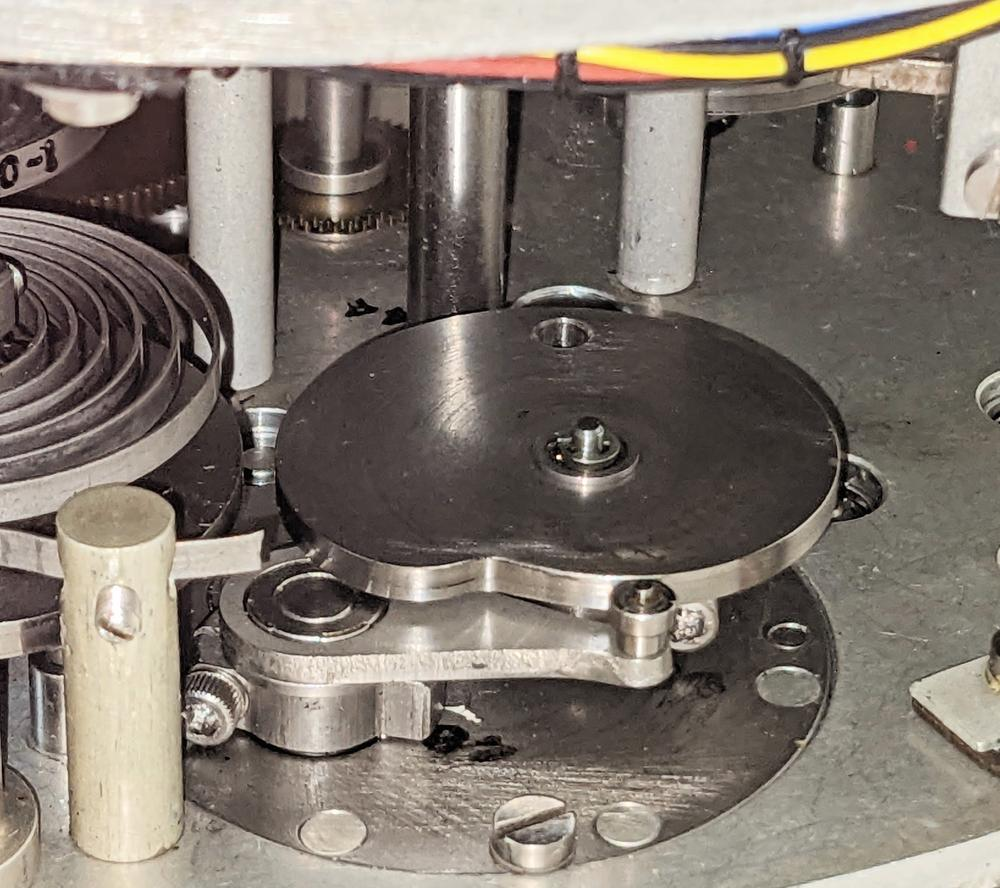A cam inside the CADC implements a function.

However, the shape of the cam doesn't provide the function directly, as you might expect. The main problem with the straightforward approach is the discontinuity when the cam wraps around, which could catch the follower. For example, if the cam implemented an exponential directly, its radius would spiral exponentially and there would be a jump back to the starting value when it wraps around.

Instead, the CADC uses a clever patented method: the cam encodes the difference between the desired function and a straight line. For example, an exponential curve is shown below (blue), with a line (red) between the endpoints. The height of the gray segment, the difference, specifies the radius of the cam (added to the cam's fixed minimum radius). The point is that this difference goes to 0 at the extremes, so the cam will no longer have a discontinuity when it wraps around. Moreover, this technique significantly reduces the size of the value (i.e. the height of the gray region is smaller than the height of the blue line), increasing the cam's accuracy.5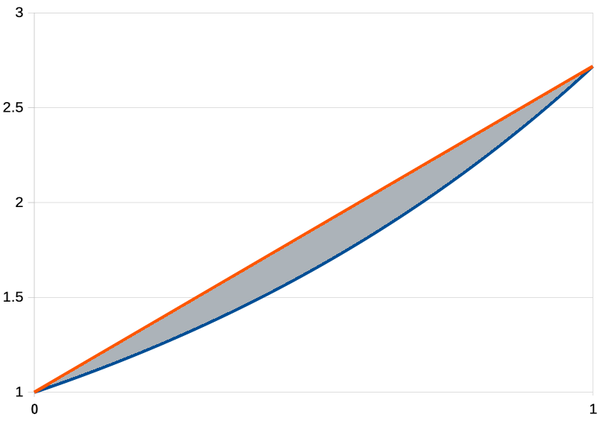An exponential curve (blue), linear curve (red), and the difference (gray).

To make this work, the cam position must be added to the linear value to yield the result. This is implemented by combining each cam with a differential gear that performs the addition or subtraction.4 As the diagram below shows, the input (23) drives the cam (30) and the differential (25, 37-41). The follower (32) tracks the cam and provides a second input (35) to the differential. The sum from the differential produces the desired function (26).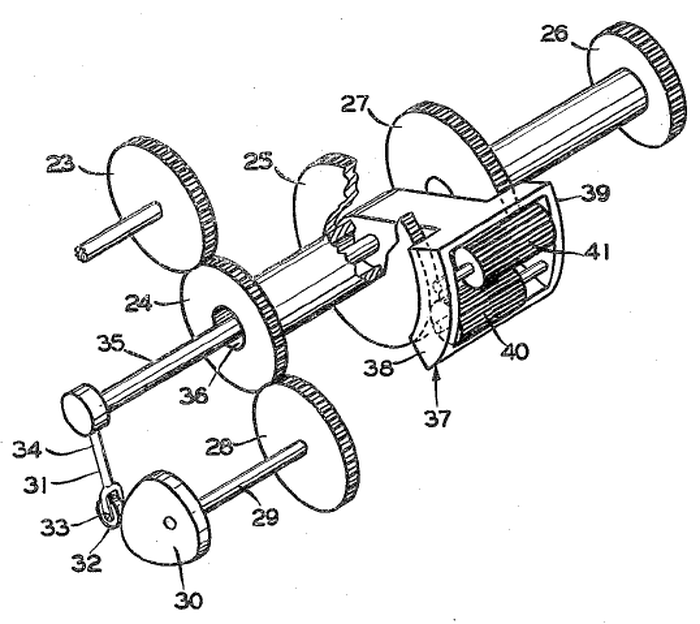This diagram, from Patent 2969910, shows how the cam and follower are connected to a differential.

### Pressure inputs

The CADC receives two pressure inputs from the pitot tube.6 Inside the CADC, two pressure transducers convert the pressures into rotational positions. Each pressure transducer contains a pair of bellows that expand and contract as the applied pressure changes. The pressure transducer has a tricky job: it must measure tiny pressure changes, but it must also provide a rotational signal that has enough torque to rotate all the gears in the CADC. To accomplish this, each pressure transducer uses a servo loop that drives a motor, controlled by a feedback loop. Cams and differentials convert the rotation into logarithmic values, providing the static pressure as $$log \; P_s$$ and the pressure ratio as $$log \; ({P_t}/{P_s})$$ to the rest of the CADC.

### The synchro outputs

A synchro is an interesting device that can transmit a rotational position electrically over three wires. In appearance, a synchro is similar to an electric motor, but its internal construction is different, as shown below. Before digital systems, synchros were very popular for transmitting signals electrically through an aircraft. For instance, a synchro could transmit an altitude reading to a cockpit display or a targeting system. Two synchros at different locations have their stator windings connected together, while the rotor windings are driven with AC. Rotating the shaft of one synchro causes the other to rotate to the same position.7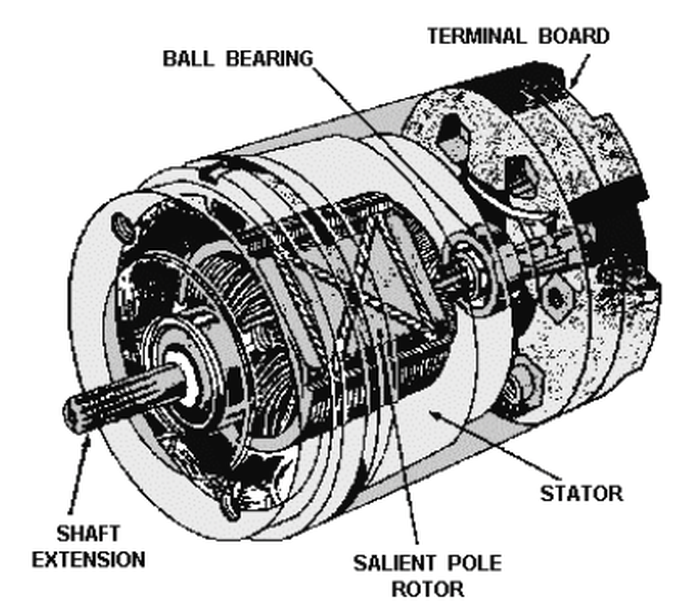Cross-section diagram of a synchro showing the rotor and stators.

For the CADC, most of the outputs are synchro signals, using compact synchros that are about 3 cm in length. For improved resolution, some of the CADC outputs use two synchros: a coarse synchro and a fine synchro. The two synchros are typically geared in an 11:1 ratio, so the fine synchro rotates 11 times as fast as the coarse synchro. Over the output range, the coarse synchro may turn 180°, providing the approximate output, while the fine synchro spins multiple times to provide more accuracy.

## Examining the left section of the CADC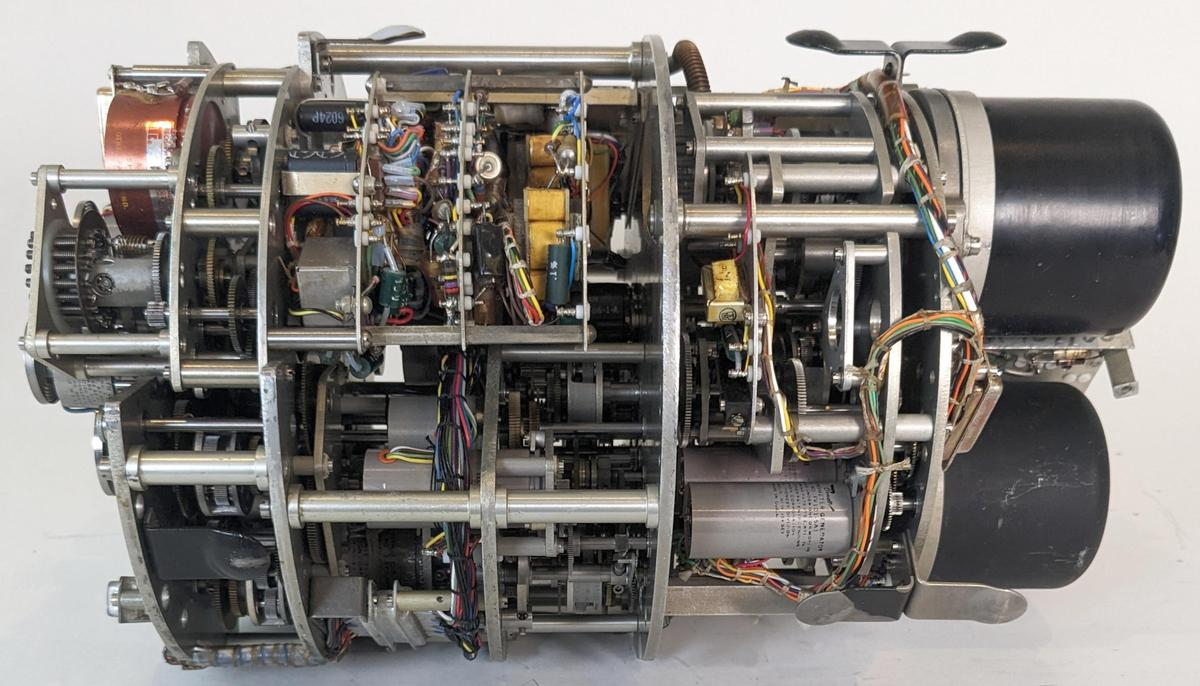The Bendix CADC is constructed from modular sections. The right section has the pressure transducers (the black domes), along with the servo mechanisms that control them. The middle section is the "Mach section". In this blog post, I'm focusing on the left section of the CADC, which computes true airspeed, air density, total temperature, log true free air temperature, and air density × speed of sound. I had feared that any attempt at disassembly would result in tiny gears flying in every direction, but the CADC was designed to be taken apart for maintenance. Thus, I could remove the left section of the CADC for analysis.

The diagram below shows the side that connects to the aircraft.8 The various synchros generate the outputs. Some of the synchros have spiral anti-backlash springs installed. These springs prevent wobble in the synchro and gear train as the gears change direction. Three of the exponential cams are visible. The differentials and gears are between the two metal plates, so they are not visible from this angle.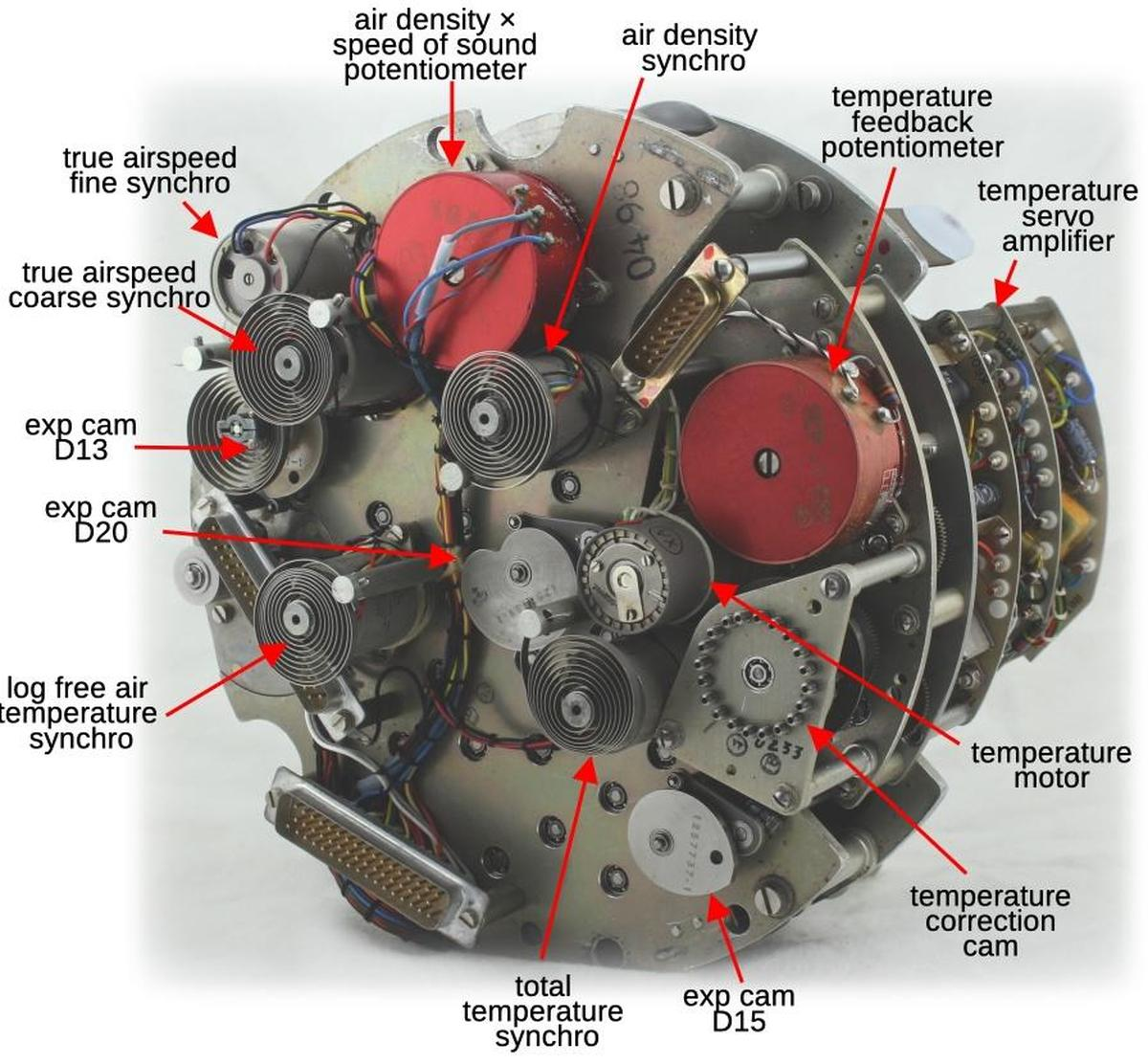The front of the CADC has multiple output synchros with anti-backlash springs.

Attached to the right side is the temperature transducer, a modular wedge that implements a motorized servo loop to convert the temperature input to a rotation. The servo amplifier consists of three boards of electronic components, including transistors and magnetic amplifiers to drive the motor. The large red potentiometer provides feedback for the servo loop. A flexible cam with 20 adjustment screws allows the transducer to be tuned to eliminate nonlinearities or other sources of error. I'll describe this module in more detail in another post.9

The photo below shows the other side of the section. This communicates with the rest of the CADC through the electrical connector and three gears that mesh with gears in the other section. Two gears receive the pressure signals $$P_t / P_s$$ and $$P_s$$ from the pressure transducer subsystem. The third gear sends the log total temperature to the rest of the CADC. The electrical connector (a standard 37-pin D-sub) supplies 120 V 400 Hz power to the rest of the CADC and passes synchro signals from the rest of the CADC to the output connectors.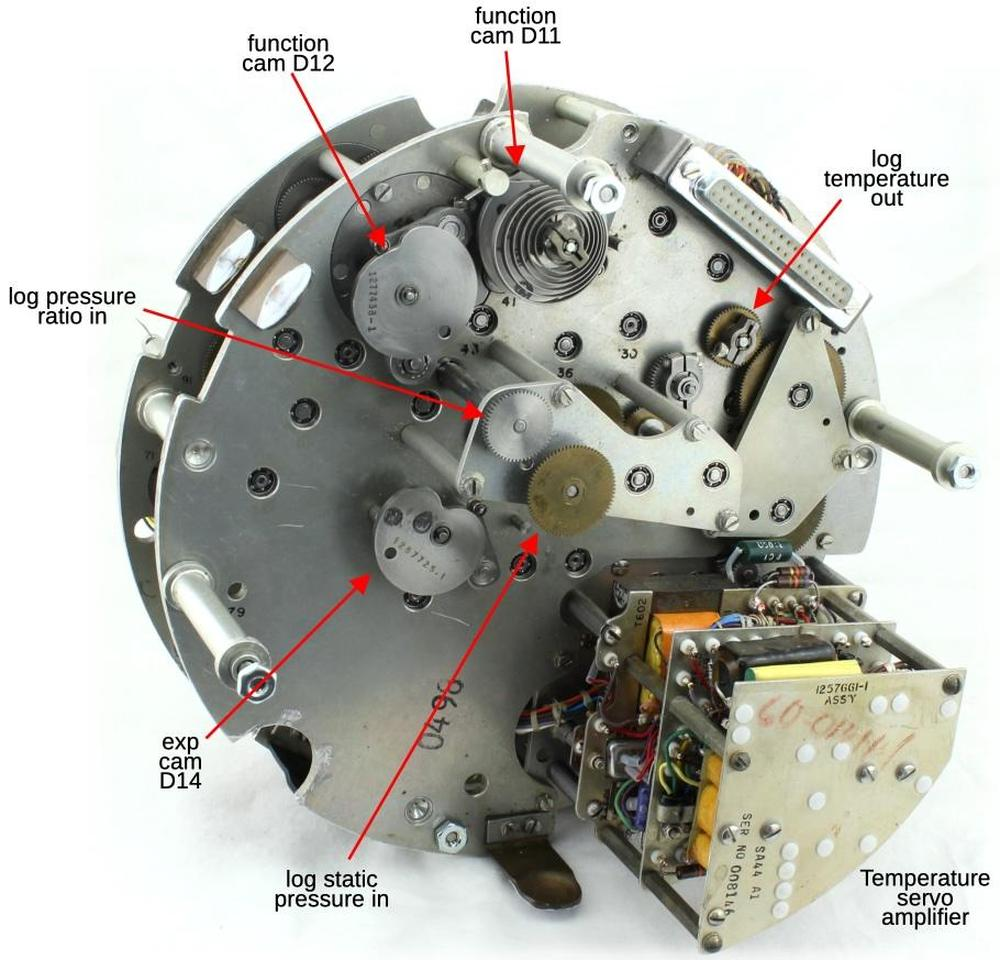This side of the section interfaces with the rest of the CADC.

## The equations

Although the CADC looks like an inscrutable conglomeration of tiny gears, it is possible to trace out the gearing and see exactly how it computes the air data functions. With considerable effort, I have reverse-engineered the mechanisms to create the diagram below, showing how each computation is broken down into mechanical steps. Each line indicates a particular value, specified by a shaft rotation. The ⊕ symbol indicates a differential gear, adding or subtracting its inputs to produce another value. The cam symbol indicates a cam coupled to a differential gear. Each cam computes either a specific function or an exponential, providing the value as a rotation. At the right, the rotations are converted to outputs, either by synchros or a potentiometer. This diagram abstracts out the physical details of the gears. In particular, scaling by constants or reversing the rotation (subtraction versus addition) are not shown.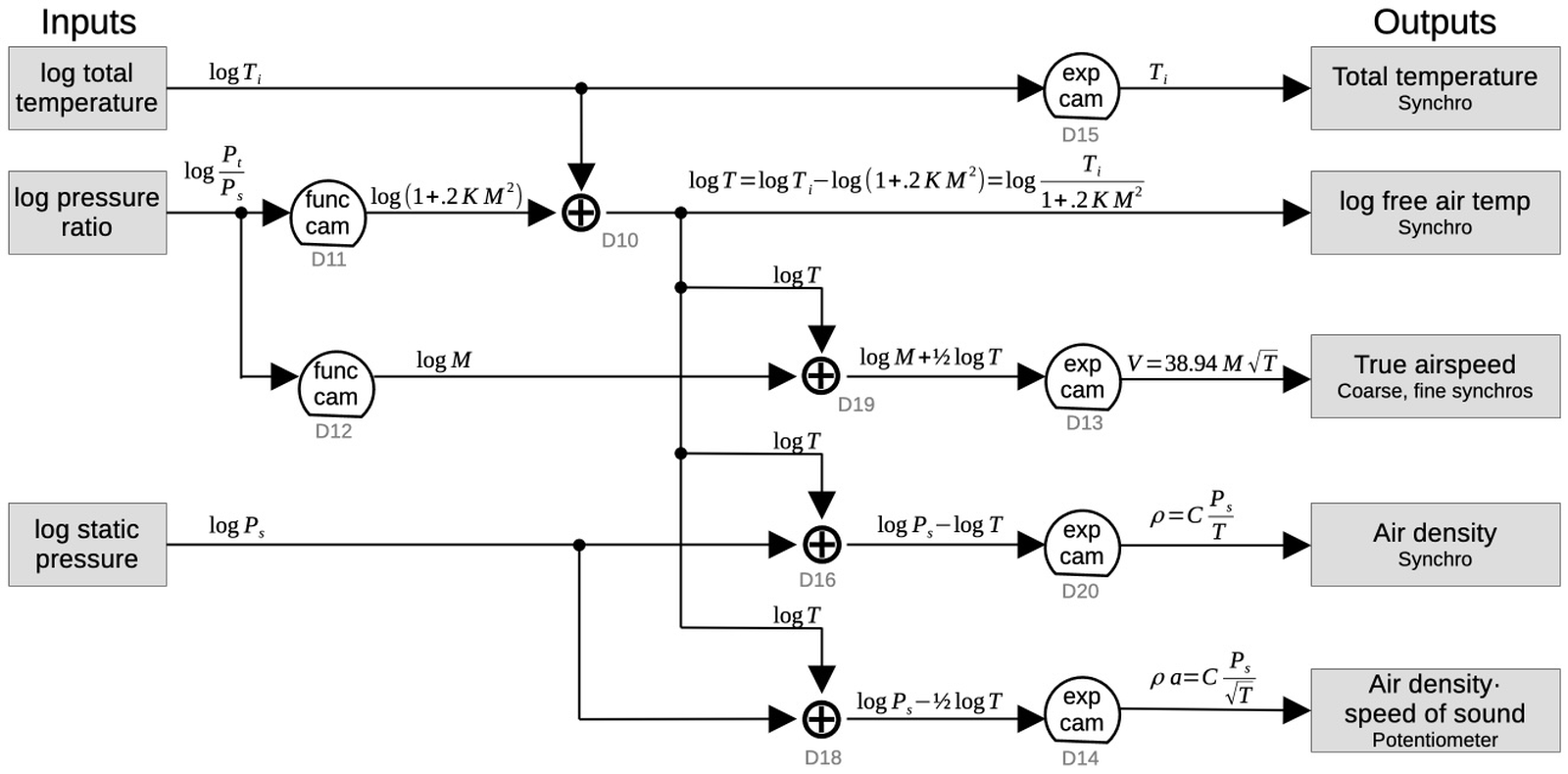This diagram shows how the values are computed. The differential numbers are my own arbitrary numbers. Click for a larger version.

I'll go through each calculation briefly.

### Total temperature

The external temperature is an important input to the CADC since it affects the air density. A platinum temperature probe provides a resistance that varies with temperature. The resistance is converted to rotation by the temperature transducer, described earlier. The definition of temperature is a bit complicated, though. The temperature outside the aircraft is called the true free air temperature, T. However, the temperature probe measures a higher temperature, called the indicated total air temperature, Ti. The reason for this discrepancy is that when the aircraft is moving at high speed, the air transfers kinetic energy to the temperature probe, heating it up.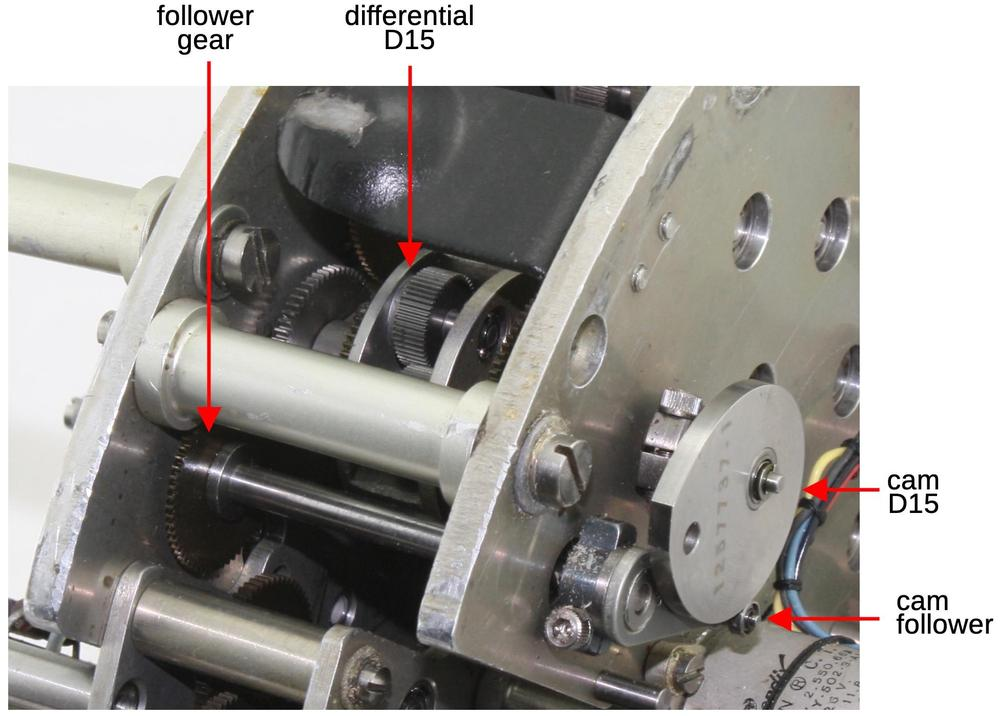The differential and cam D15.

The temperature transducer provides the log of the total temperature as a rotation. At the top of the equation diagram, cam and differential D15 simply take the exponential of this value to determine the total temperature. This rotates the shaft of a synchro to produce the total temperature as an electrical output. As shown above, the D15 cam is attached to the differential by a shaft passing through the metal plate. The follower rotates according to the cam radius, turning the follower gear which meshes with the differential input. The result from the differential is the total temperature.

### log free air temperature

A more complicated task of the CADC is to compute the true free air temperature from the measured total temperature. Free air temperature, T, is defined by the formula below, which compensates for the additional heating due to the aircraft's speed. $$T_i$$ is the indicated total temperature, M is the Mach number and K is a temperature probe constant.10

$T = \frac {T_i} {1 + .2 K M^2 }$

The diagram below shows the cams, differentials, gear trains, and synchro that compute $$log \; T$$. First, cam D11 computes $$log \; (1 + .2 K M^2 )$$. Although that expression is complicated, the key is that it is a function of one variable (M). Thus, it can be computed by cam D11, carefully shaped for this function and attached to differential D11. Differential D10 adds the log total temperature (from the temperature transducer) to produce the desired result. The indicated servo outputs this value to other aircraft systems. (Note that the output is a logarithm; it is not converted to a linear value.11 This value is also fed (via gears) into the calculations of three more equations, below.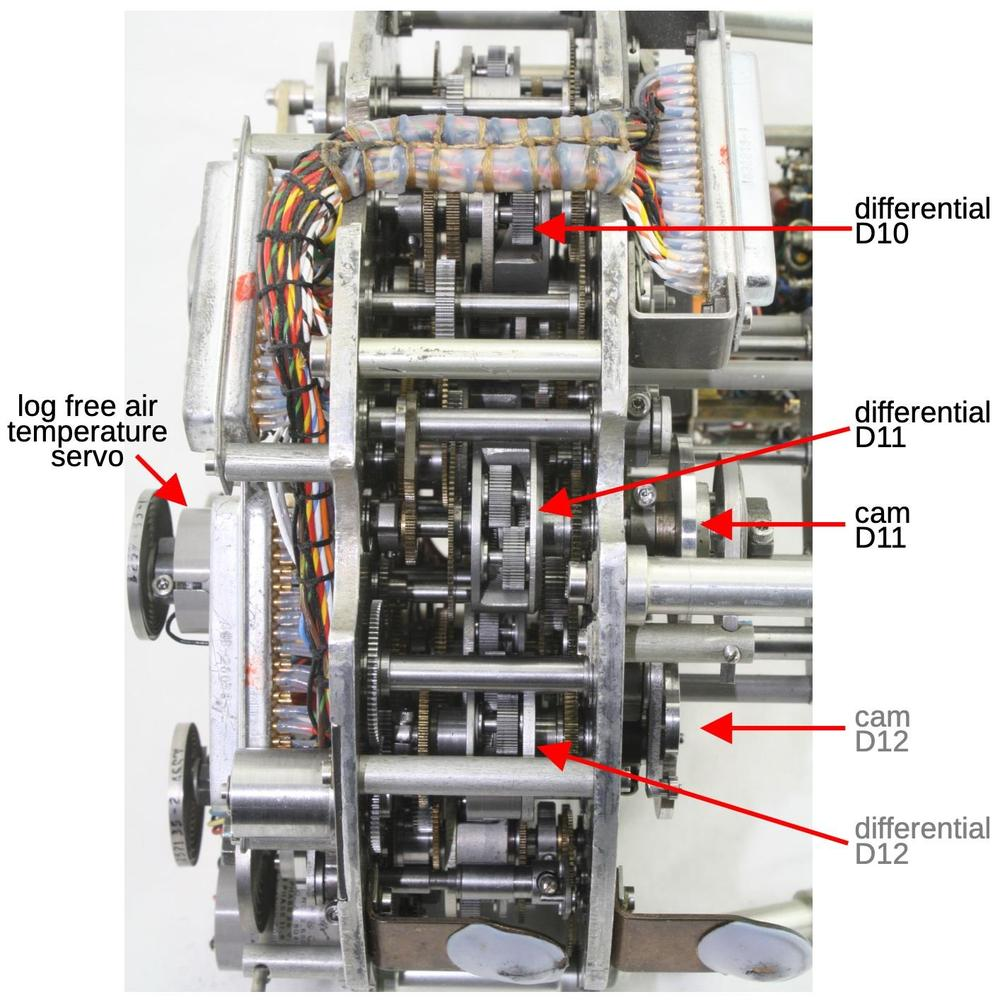The components that compute log free air temperature. D12 is not part of this equation.

### Air density

Air density is computed from the static pressure and true temperature:

$\rho = C_1 \frac{P_s} {T}$

It is calculated using logarithms. D16 subtracts the log temperature from the log pressure and cam D20 takes the exponential.

### True airspeed

True airspeed is computed from the Mach number and the total temperature according to the following formula:

$V = 38.94 M \frac{\sqrt{T_i}}{\sqrt{1+.2KM^2}}$

Substituting the true free air temperature simplifies the formula to the equation implemented in the CADC:

$V = 38.94 M \sqrt{T}$

This is computed logarithmically. First, cam and differential D12 compute $$log \; M$$ from the pressure ratio.13 Next differential D19 adds half the log temperature to multiply by the square root. Exponential cam D13 removes the logarithms, producing the final result. (The constant 38.94 is an important part of the equation, but is easily implemented with gear ratios.) The output goes to two synchros, geared to provide coarse and fine outputs.12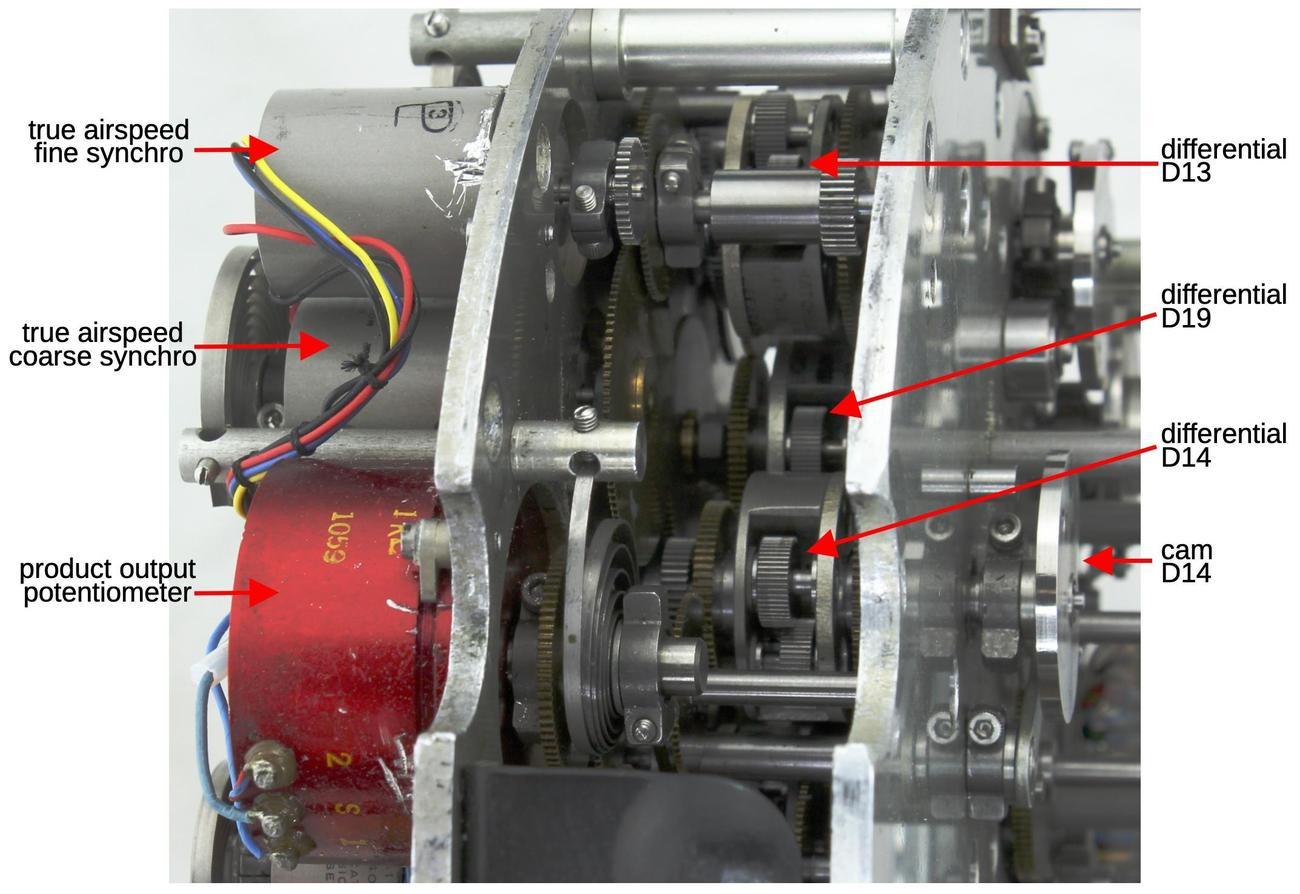These components compute true airspeed and air density × speed of sound. Note the large gear driving the coarse synchro and the small gear driving the fine synchro. This causes the fine synchro to rotate at 11 times the speed of the coarse synchro.

### Air density × speed of sound

Air density × speed of sound14 is given by the formula

$\rho \cdot a = C_2 \frac {P_s} {\sqrt{T}}$

The calculation is almost the same as the air density calculation. Differential D18 subtracts half the log temperature from the log pressure and then cam D14 computes the exponential. Unlike the other values, this output rotates the shaft of a 1 KΩ potentiometer (above), changing its resistance. I don't know why this particular value is output as a resistance rather than a synchro angle.

## Conclusions

The CADC performs nonlinear calculations that seem way too complicated to solve with mechanical gearing. But reverse-engineering the mechanism shows how the equations are broken down into steps that can be performed with cams and differentials, using logarithms for multiplication, division, and square roots. I'll point out that reverse engineering the CADC is not as easy as you might expect. It is difficult to see which gears are in contact, especially when gears are buried in the middle of the CADC and are hard to see. I did much of the reverse engineering by rotating one differential to see which other gears turn, but usually most of the gears turned due to the circuitous interconnections.15

By the late 1960s, as fighter planes became more advanced and computer technology improved, digital processors replaced the gears in air data computers. Garrett AiResearch's ILAAS air data computer (1967) was the first all-digital unit. Other digital systems were Bendix's ADC-1000 Digital Air Data Computer (1967) which was "designed to solve all air data computations at a rate of 75 times per second", Conrac's 3-pound solid-state air data computer (1967), Honeywell's Digital Air Data System (1968), and the LSI-based Garrett AiResearch F-14 CADC (1970). Nonetheless, the gear-based Bendix CADC provides an interesting reverse-engineering challenge as well as a look at the forgotten era of analog computing.

For more background on the CADC, see my overview article on the CADC. I plan to continue reverse-engineering the Bendix CADC and get it operational,16 so follow me on Twitter @kenshirriff or RSS for updates. I've also started experimenting with Mastodon as @oldbytes.space@kenshirriff. Thanks to Joe for providing the CADC. Thanks to Nancy Chen for obtaining a hard-to-find document for me. Marc Verdiell and Eric Schlaepfer are working on the CADC with me.

## Notes and references

1. The static air pressure can also be provided by holes in the side of the pitot tube. I couldn't find information indicating exactly how the planes with the CADC received static pressure.

2. Although the CADC's equations may seem ad hoc, they can be derived from fluid dynamics principles. These equations were standardized in the 1950s by various government organizations including the National Bureau of Standards and NACA (the precursor of NASA).

3. Strictly speaking, the output of the differential is the sum of the inputs divided by two. I'm ignoring the factor of 2 because the gear ratios can easily cancel it out. It's also arbitrary whether you think of the differential as adding or subtracting, since it depends on which rotation direction is defined as positive.

4. The cam value will be added or subtracted, depending on whether the function is concave or convex. This is a simple matter of gearing when the values are fed into the differential. Matching the linear segment to the function is also done with gearing that scales the input value appropriately.

5. The diagram below shows a typical cam function in more detail. The input is $$log~ dP/P_s$$ and the output is $$log~M / \sqrt{1+.2KM^2}$$. The small humped curve at the bottom is the cam correction. Although the input and output functions cover a wide range, the difference that is encoded in the cam is much smaller and drops to zero at both ends.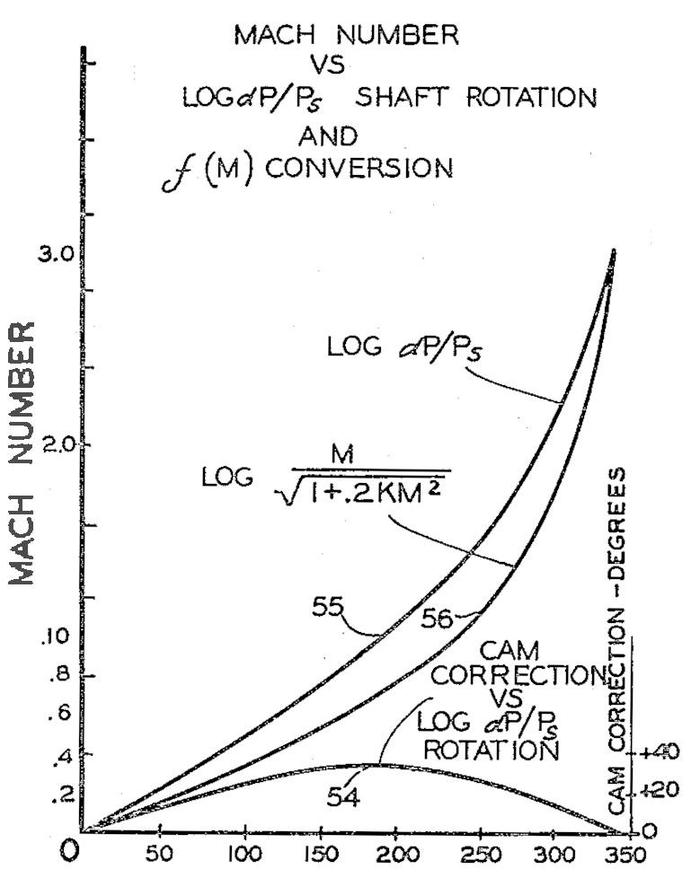This diagram, from Patent 2969910, shows how a cam implements a complicated function.

6. The CADC also has an input for the "position error correction", which I will ignore in this post. This input provides a correction factor because the measured static pressure may not exactly match the real static pressure. The problem is that the static pressure is measured from a port on the aircraft. Distortions in the airflow may cause errors in this measurement. A separate box, the "compensator", determined the correction factor based on the angle of attack and fed it to the CADC as a synchro signal.

7. Internally, a synchro has a moving rotor winding and three fixed stator windings. When AC is applied to the rotor, voltages are developed on the stator windings depending on the position of the rotor. These voltages produce a torque that rotates the synchros to the same position. In other words, the rotor receives power (26 V, 400 Hz in this case), while the three stator wires transmit the position. The diagram below shows how a synchro is represented schematically, with rotor and stator coils.

The schematic symbol for a synchro.

8. The CADC is wired to the rest of the aircraft through round military connectors. The front panel interfaces these connectors to the D-sub connectors used internally. The two pressure inputs are the black cylinders at the bottom of the photo.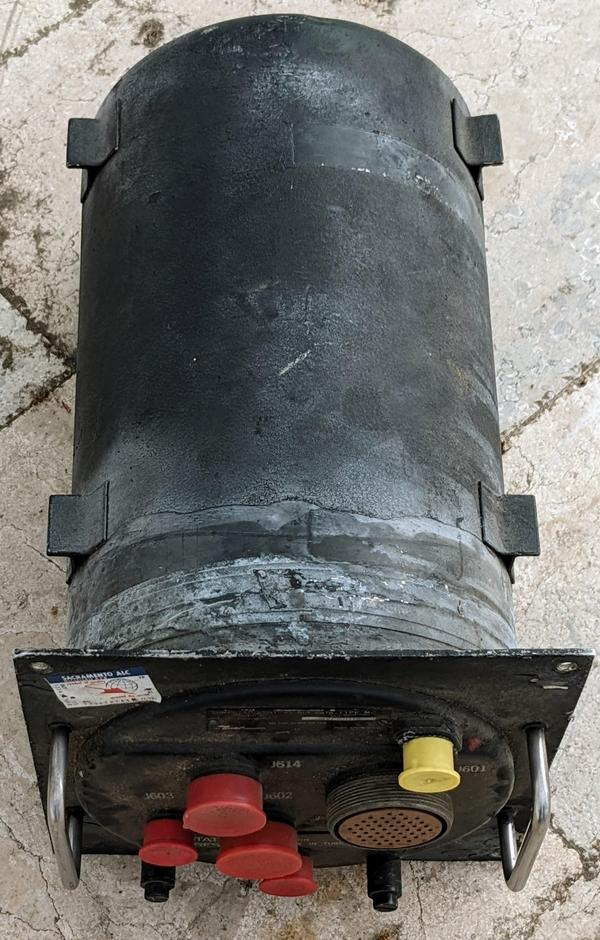The exterior of the CADC. It is packaged in a rugged metal cylinder.

9. I don't have a blog post on the temperature module yet, but I have a description on Twitter and a video

10. The constant K depends on the recovery factor of the temperature probe. This compensates for a probe where not all of the air's kinetic energy gets transferred to the probe. The 1958 description says that with "modern total temperature probes available today", the K factor can be considered to be 1.

11. The CADC specification says that it provides the log true free air temperature from -80° to +70° C. Obviously the log won't work for a negative value so I assume this is the log of the Kelvin temperature (°K).

12. The CADC specification defines how the parameter values correspond to rotation angles of the synchros. For instance, for the airspeed synchros, the CADC supports the airspeed range 104.3 to 1864.7 knots. The coarse and fine outputs are geared in an 11:1 ratio, so the fine synchro will rotate multiple times over the range to provide more accuracy. Over this range, the coarse synchro rotates from -18.94° to +151.42° and the fine synchro rotates from -208.29° to +1665.68°, with 0° corresponding to 300 knots.

13. The Mach function is defined in terms of $$P_t/P_s$$, with separate cases for subsonic and supersonic:

$M<1:$ $~~~\frac{P_t}{P_s} = ( 1+.2M^2)^{3.5}$

$M > 1:$

$~~~\frac{P_t}{P_s} = \frac{166.9215M^7}{( 7M^2-1)^{2.5}}$

Although these equations are very complicated, the solution is a function of one variable $$P_t/P_s$$ so M can be computed with a single cam. In other words, the mathematics needed to be done when the CADC was manufactured, but once the cam exists, computing M is trivial.

14. I'm not sure why the CADC computes air density times speed of sound. I couldn't find any useful aircraft characteristics that depend on this value, but there must be something. In acoustics and audio, this product is useful as the "air impedance", but I couldn't determine the relevance for aviation.

15. While reverse-engineering this system, I have gained more appreciation for the engineering involved. Converting complicated equations to gearing is a remarkable feat. But also remarkable is designing the CADC as a three-dimensional object that can be built, disassembled, and repaired, long before any sort of 3-D modeling was available. It must have been a puzzle to figure out where to position each differential. Each differential had three gears driving it, which had to mesh with gears from other differentials. There wasn't much flexibility in the gear dimensions, since the gear ratios had to be correct and the number of teeth on each gear had to be an integer. Moreover, it is impressive how tightly the gears are packed together without conflicting with each other.

16. It was very difficult to find information about the CADC. The official military specification is MIL-C-25653C(USAF). After searching everywhere, I was finally able to get a copy from the Technical Reports & Standards unit of the Library of Congress. The other useful document was in an obscure conference proceedings from 1958: "Air Data Computer Mechanization" (Hazen), Symposium on the USAF Flight Control Data Integration Program, Wright Air Dev Center US Air Force, Feb 3-4, 1958, pp 171-194.

Hangar Eighteen said...

I found a lot of similar calculations in a document named "Fixed Wing Performance" and serialized as USNTPS-FTM-108. It contains many of the original calculations and their simplifications for use in this application.

Jeff said...

Ken, how similar is this electromechanical computer to the Ford Instrument Company Mark I Fire Control Computer? That device has cams that look superficially similar (to my completely untrained eye). I was surprised to see the patent granted to Bendix in 1961 when it seemed that there was obvious prior art.

Dr Jaymz said...

I know that its a very large collection of well known engineering concepts and that they can turn the handle on the requirements and get the outputs and design they want. But its still mindboggling that its in that single can and its all fits together. Arranging a method to test it is even more mindboggling. Its perhaps overlooked that when developing fighters in the 1950s there would be thousands in well lit rooms consuming lots of coffee with a thick haze of smoke working on such things using mostly paper and slide rules. To bring it altogether in any timely fashion, I find it amazing. The number of man hours in that thing must be very very large as is its cost. I built a hobbyist flight data computer that provides AHRS, angle of attack, measure static and pitot pressure and OAT and it can all be done on a single ESP32. Along the way I found everything I needed, formulas, concepts etc all published in the 40's and 50's. They did a great job, I wouldn't have stood a chance trying to get my head around any part of it in the 1950s.Dr. Peter said...

Those were REAL engineers.Anonymous said...

I believe our thinking now has become straight jacketed, due to ubiquitous use of the microprocessor.
The possibility of other solutions rarely even comes into our mind now.
This ingenious device is rad hard too, and is serviceable decades after its planned life. Yet today we would design this
With a dozen processors or fpgas